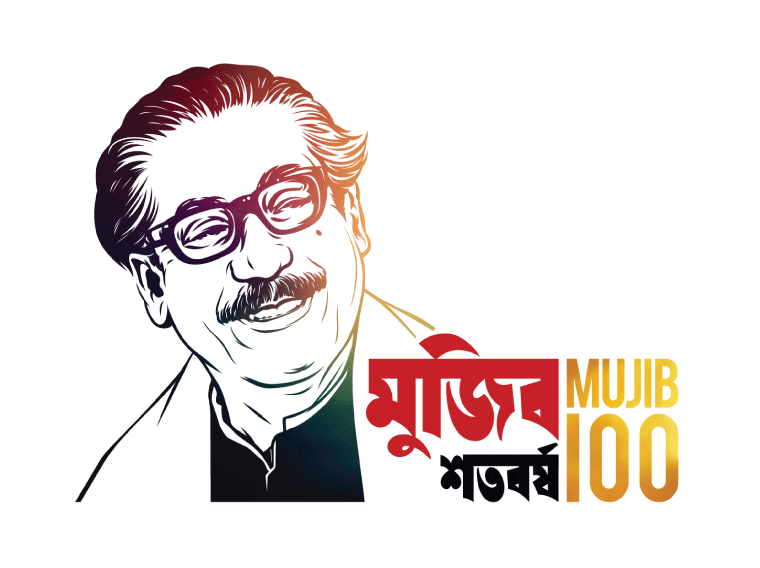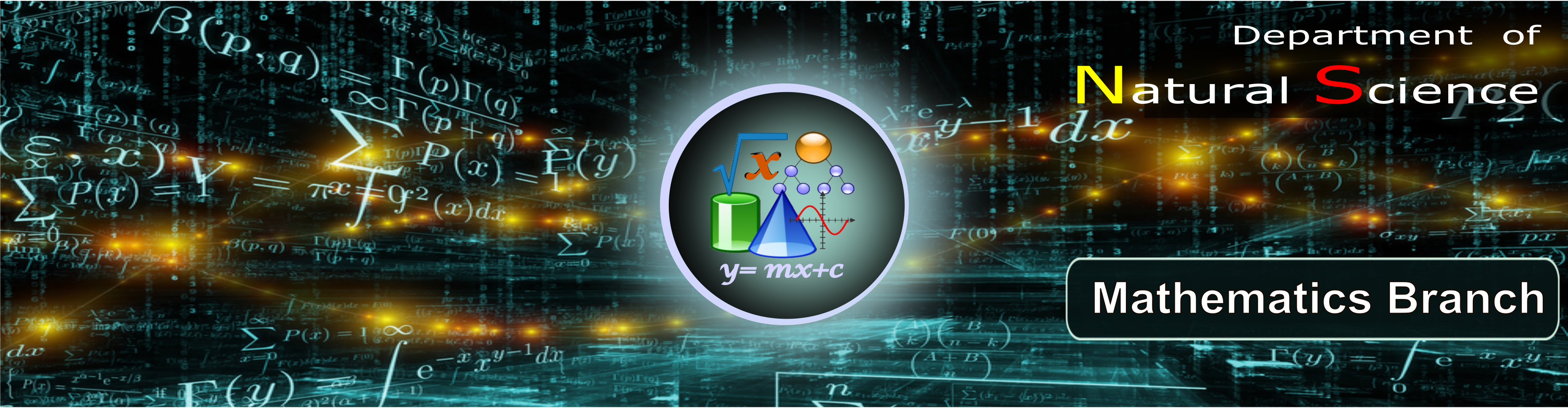Welcome to Port City International University#### Course of mathematics

Mathematics has been fundamental to the development of science and technology. In recent decades, the extent of application of mathematics to real-world problems has increased by leaps and bounds. The present syllabi of B.Sc. have been designed as per the U.G.C Model curriculum so that students learn basic concepts of Mathematics and are exposed to rigorous methods gently and slowly.

MAIN COURSES:

1. Complex Variable and Laplace Transformation
2. Differential Equations and Fourier Analysis
3. Linear Algebra
4. Matrices and Three Dimensional Coordinate Geometry
5. Differential and Integral Calculus
6. Vector Analysis
7. Numerical Analysis
9. Differential Calculus and Coordinate Geometry

GOALS: Give the students a sufficient knowledge of fundamental principles, methods and a clear perception of innumerous power of mathematical ideas and tools and know how to use them by modeling, solving and interpreting.

OBJECTIVE: Enhancing students overall development and to equip them with mathematical modelingabilities, problem solving skills, creative talent and power of communication necessary or various kinds of employment.

#### Teachers

View Teacher List of Course of mathematics